# How to Round a Range of Cells in Excel

This post will guide you how to round a range of cells with a formula in Excel. How do I round a range of cells using VBA macro in Excel 2013/2016. How to round up a range of numbers in a column in Excel.

## Round a Range of Cells with ROUND Function

Assuming that you have a list of data in range B1:B5 which contain numbers with several decimal places. And you want to round them with only two decimal places. You can use a formula based on the ROUND function to achieve the result. Like as follows:

`=ROUND(B1,2)`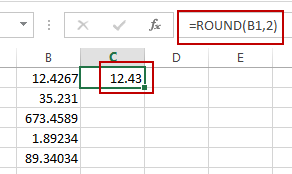Then you need to type the formula in a blank cell and drag the AutoFill handle over to other cells to apply this formula.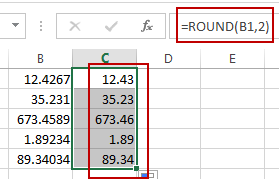## ROUND a Range of Cells with VBA Macro

You can also use an Excel VBA Macro to achieve the same result of rounding a range of cells quickly in Excel. Just do the following steps:

#1 open your excel workbook and then click on “Visual Basic” command under DEVELOPER Tab, or just press “ALT+F11” shortcut.#2 then the “Visual Basic Editor” window will appear.

#3 click “Insert” ->”Module” to create a new module.#4 paste the below VBA code into the code window. Then clicking “Save” button.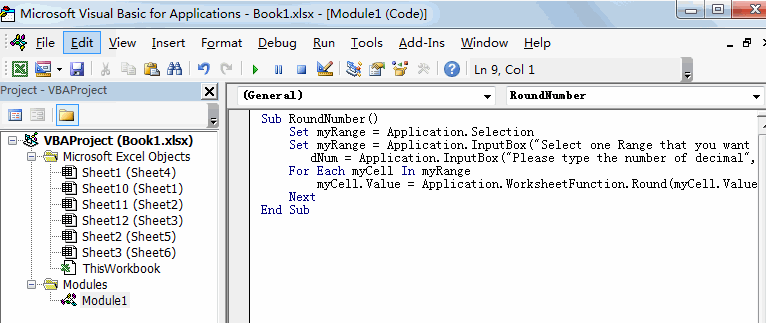```Sub RoundNumber()
Set myRange = Application.Selection
Set myRange = Application.InputBox("Select one Range that you want to round up", "RoundNumber", myRange.Address, Type:=8)
dNum = Application.InputBox("Please type the number of decimal", "RoundNumber", Type:=1)
For Each myCell In myRange
myCell.Value = Application.WorksheetFunction.Round(myCell.Value, dNum)
Next
End Sub```

#5 back to the current worksheet, then run the above excel macro. Click Run button.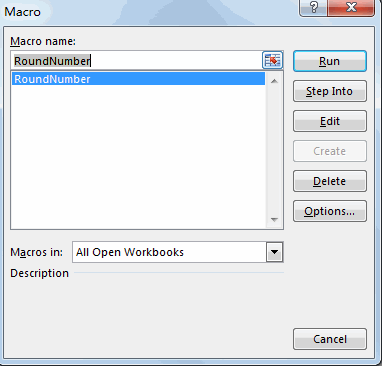#6 Select one Range that you want to round up, such as: B1:B5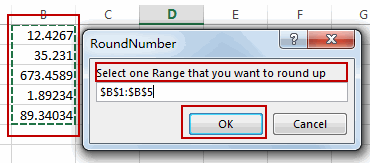#7 Please type the number of decimal that you want to round.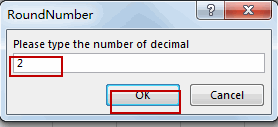#8 Let’s see the last result: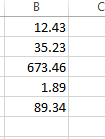Video: Round a Range of Cells

### Related Functions

• Excel Round function
The Excel INT function rounds a number to a specified number of digits. You can use the ROUND function to round to the left or right of the decimal point in Excel.The syntax of the ROUND function is as below:=ROUND (number, num_digits)…